What is

What is the value of the smaller of a pair of numbers for which their sum is 78 and their division quotients are 0.3?

Result

a =  18

Solution:Leave us a comment of example and its solution (i.e. if it is still somewhat unclear...):Be the first to comment!To solve this example are needed these knowledge from mathematics:

Do you have a linear equation or system of equations and looking for its solution? Or do you have quadratic equation?

Next similar examples:

1. Double 5Peter was thinking of a number. Peter doubles it and gets an answer of 8.6. What was the original number?
2. Number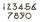What number I think? The third is 6 and half is 2 more than the its quarter.
3. MultiplesFind all multiples of 10 that are larger than 136 and smaller than 214.
4. ProductResult of the product of the numbers 1, 2, 3, 1, 2, 0 is:
5. Expression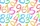If A=2 B=3 evaluate expression A(B+A) and multiply it by A
6. NumberCalculate the integer number which, divided by 34 gives 10 and the rest 25.
7. Products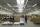15 products are 48 € more expensive than 12 products. How many euros will it cost five same products?
8. Women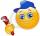In an company of 1050 employees are 2/3 women. How many women work in the company?
9. DoctorsIn the city operates 171 doctors. The city has 128934 citizens. How many citizens are per one doctor?
10. Salary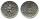Mr. Vesely got for work 874 CZK (Czech Republic Koruna). Mr. Jaros got twice less than Mr. Vesely. How much CZK got Mr. Jaros?
11. Bakers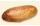Baker Martin baked 5 times more cakes than Dennis. How many cakes baked Dennis if Martin bake 25 cakes?
12. Family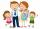Dad has two years more than mom. Mom has 5 times more than Katy. Katy has 2 times less than Jan. Jan is 10 years old. How old is everyone in the family? How old are all together?
13. Balls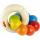The boys changed stamps, beads and balls. For 9 balls is 3 stamps, 2 balls is 44 stamps. How many beads is for 1 ball?
14. Rolls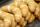Mom every day buys 6 rolls. On Friday buys 2 times as much. How many rolls buys on Friday?
15. Simple equationSolve for x: 3(x + 2) = x - 18
16. Chestnuts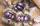Neil has 5-times chestnuts less than Adrian. Together they have 894 chestnuts. How many chestnuts has Neil and Adrian?
17. Forest nurseryIn the forest nursery after winter, they found that 1/10 stems died out of them. For them, they land 193 new spruces. How many spruces are in the forest nursery?